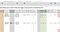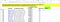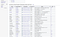# Useful Google Sheets Functions for OSINT research

When conducting a large open source research project, you want a way to store your data and share it with collaborators. My tool of choice for this is Google Sheets because it has real-time collaboration and allows for easy tracking of changes.

Google Sheets operates much like Microsoft’s Excel, if you are familiar with one, you’ll find the other a breeze to use.

I’m aware that this is one of the least glamourous parts of an OSINT investigation, but I do hope you can learn some useful tricks here. Please feel free to get in touch with me on Twitter if you have any others to add @tomtomjarvis.

Much of this guide will look at the tools used in the Tibet Research Project, an open source research project documenting prisons and detention centres in Tibet and the surrounding regions to provide a foundation for future human rights research.

This research involves the location, analysis, classification, and identification of prisons in the regions of interest and so a large amount of data is generated.

One of the best tools when researching foreign countries is the Google Translate function in Google Sheets. This allows you to generate translations for text in cells which can be useful when searching for information.

In my case, I had a list of counties to search and wanted to generate the names in Chinese and generate Baidu search links for easy access to information. (For those of you unfamiliar with Chinese internet, Baidu is their equivalent of Google).

To use the Google Translate function, you need to know several things. Firstly the function:

Then within that, you want the cell that needs to be translated, the language you use, and the language you are translating to. To tell Sheets what languages you are using, you put the two-letter codes in brackets. You can find the two-letter codes with this link.

The above function will translate the contents of cell C3 from English (“en”) to Chinese (“zh”).

Generating hyperlinks ranges from easy to hard depending on how websites use their links. Some sites are fairly straightforward, others are much more complicated.

The website Satellites.Pro has a sensible URL structure, for example.

https://satellites.pro/China_map#29.691272,91.157717,19

It has its basic web link, followed by the region and the decimal coordinates, then a zoom factor.

If you know the country and the coordinates you can generate the link:

satellites.pro/China_map#[LATITUDE],[LONGITUDE],19

As seen above we want to insert the latitude and longitude into this weblink. We could also change the final number, 19, if we want to change the zoom factor.

To generate this link from the coordinates, we just need two functions:The HYPERLINK function allows you to generate links from values such as coordinates or search terms. Seen above are the links to mapping software based on a row’s coordinates.

HYPERLINK allows you to make a link from a URL in a cell and defines the text that shows as the clickable link.

CONCATENATE allows you to join cells together, useful when joining the link structure with the coordinates.

Firstly, let’s generate the URL we want.

=CONCATENATE(“https://satellites.pro/#”, N7, “,18”)

This connects the value of cell N7 which is our coordinates cell to the end of the URL, then adds the string “,18” at the end to define the zoom factor.

the result would be:

https://satellites.pro/#[COORDINATES],18

Combining these gives us the formula:

This can also be used for the first example where we translated names into search terms in a search engine such as Baidu.Baidu links generated in the same manner. The search term is appended onto the URL. It took me some time to figure out what URL structure worked here and there may be a shorter way of doing it — if so, let me know!

# Splitting Coordinates into Latitude and Longitude

To separate coordinates from a decimal value into latitude and longitude values in Google Sheets, you can use the TRIM, INDEX, and SPLIT functions. What these do is split the string at a defined point — in this case, the comma. Then it takes the first or second part as defined by the user, then gets rid of the rest.

For Latitude

=IFERROR(TRIM(INDEX(split(N7, “,”),1)))

For Longitude

=IFERROR(TRIM(INDEX(split(N7, “,”),2)))

(Where N7 is the cell containing the decimal coordinates)

How does this work? Let’s break down the formula. The sections in bold are the ones we look at.

=IFERROR(TRIM(INDEX(split(N7, “,”),1)))

Firstly the SPLIT function is told what cell to look at, then splits it at the part in quote marks. In this case, we are telling Sheets to split at the comma.

=IFERROR(TRIM(INDEX(split(N7, “,”),1)))

Then the INDEX function uses that and indexes either the first or second part. Here you can see the number is 1, meaning everything before the comma is used. If you wanted everything after the comma, you would use the number 2.

=IFERROR(TRIM(INDEX(split(N7, “,”),1)))

Finally, the TRIM function gets rid of the rest. You will also see an IFERROR function. This is just a handy tool to wrap around other functions to allow for a blank cell or an error message if something fails.

# Data Scraping

Data scraping is a great way of copying data into your spreadsheet from web pages. It is a little more complicated than some of the above options and is a bit more fiddly, so for a full overview, check out my full guide here.Scraping functions can allow you to import tables from sites such as Wikipedia

As seen in the image above, a useful starting point is Wikipedia tables which are often easiest to scrape. This table is of Chinese air force bases and is a great example because it contains coordinates and can be imported into a Google Map from Google Sheets to visually map the locations.

The code needed to scrape data from the PLA airbases page is a simple Import HTML formula.

=ImportHtml(“https://en.wikipedia.org/wiki/List_of_People%27s_Liberation_Army_Air_Force_airbases", “table”, 6)

This works by defining the URL to scrape, then the data type (table), then which table to use (6).

In the above example, the table we wanted was the sixth on the page, so we added the 6 value. Sometimes this can be a little hard to determine so playing about is often necessary.

Again, I highly recommend you check out my full guide on this one, as there is a lot of useful information covered.

# Stripping Units from data

Sometimes you have values added which have units. An example of this is the prison perimeter column in the Tibet Research Project’s researcher dataset.

Values here are followed by an “m” for metres, e.g "500 m”. This can be a problem if wanting to calculate values or generate a report on, say, the total perimeter distance of all prisons found. To solve this we need to strip out the units characters.

To do this, we can use the following formula:

=IFERROR(LEFT((SUBSTITUTE(AA7, “,”, “”, 1)), LEN(AA7)-2))

First, we look inside the innermost brackets, which is the substitute function. This will substitute all non-numbers with “” (nothing).

Next, we figure out how many characters there are to be removed (in this case, two) and remove them with the LEFT function. The LEN function just determines the length of the full string.

Put simply, we are picking the first X number of characters, where X is the original length minus two (the two deleted characters which include a space).